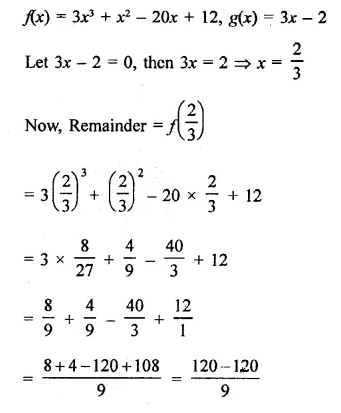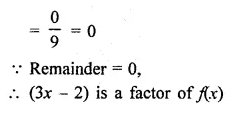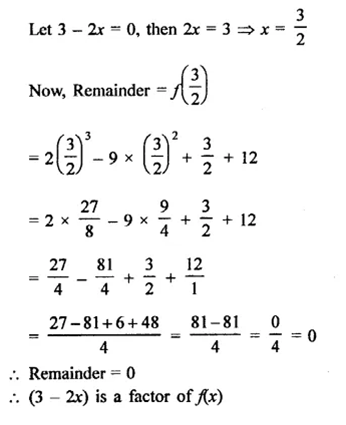• +91 9971497814
• info@interviewmaterial.com

# RD Chapter 6- Factorisation of Polynomials Ex-6.4 Interview Questions Answers

### Related Subjects

Question 1 : In each of the following, use factor Theorem to findwhether polynomial g(x) is a factor of polynomial f(x) or, not: (1-7)
f(x) = x3 – 6x2 + 11x– 6; g(x) = x – 3

We know that if g(x) is a factor of p(x),
then the remainder will be zero. Now,
f(x) = x3 – 6x2 + 11x – 6; g(x) = x -3
Let x – 3 = 0, then x = 3
Remainder = f(3)
= (3)3 – 6(3)2 +11 x 3 – 6
= 27-54 + 33 -6
= 60 – 60 – 0
Remainder is zero,
x – 3is a factor of f(x)

Question 2 : f(x) = 3X4 + 17x3 + 9x2 –7x – 10; g(x) = x + 5

f(x) = 3x4 + 17X3 + 9x2 –7x – 10; g(x) = x + 5
Let x + 5 = 0, then x = -5
Remainder = f(-5) = 3(-5)4 + 17(-5)3 + 9(-5)2 –7(-5) – 10
= 3 x 625 + 17 x (-125) + 9 x (25) – 7 x (-5) – 10
= 1875 -2125 + 225 + 35 – 10
= 2135 – 2135 = 0
Remainder = 0
(x +5) is a factor of f(x)

Question 3 : f(x) = x5 + 3x4 – x3 –3x2 + 5x + 15, g(x) = x + 3

f(x) = x5 + 3X4 – x3 –3x2 + 5x + 15, g(x) = x + 3
Let x + 3 = 0, then x = -3
Remainder = f(-3)
= (-3)5 + 3(-3)4 – (-3)3 –3(-3)2 + 5(-3) + 15
= -243 + 3 x 81 -(-27)-3 x 9 + 5(-3) + 15
= -243 +243 + 27-27- 15 + 15
= 285 – 285 = 0
Remainder = 0
(x + 3) is a factor of f(x)

Question 4 : f(x) = x3 – 6x2 – 19x+ 84, g(x) = x – 7

f(x) = x3 – 6x2 – 19x + 84, g(x)= x – 7
Let x – 7 = 0, then x = 7
Remainder = f(7)
= (7)3 – 6(7)2 – 19 x 7 + 84
= 343 – 294 – 133 + 84
= 343 + 84 – 294 – 133
= 427 – 427 = 0
Remainder = 0
(x– 7) is a factor of f(x)

Question 5 : f(x) = 3x3  + x2 –20x + 12, g(x) = 3x – 2Question 6 : f(x) = 2x3 – 9x2 + x +12, g(x) = 3 – 2x

Answer 6 : f(x) = 2x3 – 9x2 + x +12, g(x) = 3 – 2xQuestion 7 : f(x) = x3 – 6x2 + 11x– 6, g(x) = x2 – 3x + 2

g(x) = x2 – 3x + 2
= x2 – x – 2x + 2
= x(x – 1) – 2(x – 1)
= (x – 1) (x – 2)
If x – 1 = 0, then x = 1
f(1) =(1)3 – 6(1)2 + 11(1) – 6
= 1-6+11-6= 12- 12 = 0
Remainder is zero
x – 1is a factor of f(x)
and if x – 2 = 0, then x = 2
f(2) =(2)3 – 6(2)2 + 11(2)-6
= 8 – 24 + 22 – 6 = 30 – 30 = 0
Remainder = 0
x – 2is also a factor of f(x)

Question 8 : Show that (x – 2), (x + 3) and (x – 4) are factors ofx3 – 3x2 – 10x + 24.

f(x) = x3 – 3x2 – 10x + 24
Let x – 2 = 0, then x = 2
Now f(2) = (2)3 – 3(2)2 – 10 x 2 + 24
= 8 – 12 – 20 + 24 = 32 – 32 = 0
Remainder = 0
(x –2) is the factor of f(x)
If x + 3 = 0, then x = -3
Now, f(-3) = (-3)3 – 3(-3)2 – 10 (-3) + 24
= -27 -27 + 30 + 24
= -54 + 54 = 0
Remainder = 0
(x +3) is a factor of f(x)
If x – 4 = 0, then x = 4
Now f(4) = (4)3 – 3(4)2 – 10 x 4 + 24 = 64-48-40 + 24
= 88 – 88 = 0
Remainder = 0
(x –4) is a factor of (x)
Hence (x – 2), (x + 3) and (x – 4) are the factors of f(x)

Question 9 : Show that (x + 4), (x – 3) and (x – 7) are factors ofx3 – 6x2 – 19x + 84.

Let f(x) = x3 – 6x2 – 19x + 84
If x + 4 = 0, then x = -4
Now, f(-4) = (-4)3 – 6(-4)2 – 19(-4) + 84
= -64 – 96 + 76 + 84
= 160 – 160 = 0
Remainder = 0
(x +4) is a factor of f(x)
If x – 3 = 0, then x = 3
Now, f(3) = (3)3 – 6(3)2 – 19 x 3 + 84
= 27 – 54 – 57 + 84
= 111 -111=0
Remainder = 0
(x –3) is a factor of f(x)
and if x – 7 = 0, then x = 7
Now, f(7) = (7)3 – 6(7)2 – 19 x 7 + 84
= 343 – 294 – 133 + 84
= 427 – 427 = 0
Remainder = 0
(x –7) is also a factor of f(x)
Hence (x + 4), (x – 3), (x – 7) are the factors of f(x)

Question 10 : For what value of a (x – 5) is a factor of x3 –3x2 + ax – 10?

f(x) =x3 – 3x2 + ax – 10
Let x – 5 = 0, then x = 5
Now, f(5) = (5)3 – 3(5)2 + a x 5 – 10
= 125 – 75 + 5a – 10
= 125 – 85 + 5a = 40 + 5a
(x –5) is a factor of fix)
Remainder = 0
40 + 5a = 0  5a = -40
a= 405= -8
Hence a = -8

Todays Deals### RD Chapter 6- Factorisation of Polynomials Ex-6.4 Contributorskrishan

Name:
Email:

# Latest News# 9000 interview questions in different categories